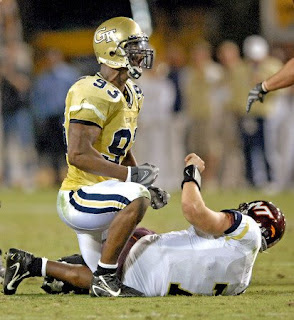## Tuesday, April 15, 2008

### Engineers Doing Productive Work

Now, I call this productive work. "Texaspetegt" at the Hive calls this the "Bobby Dodd Stadium Visual Acuity Tracker". It's an Excel spreadsheet with BDS mapped on it. Click on any section and see how good your view of the field is, based on that class you took where you learned about the Cartesian Coordinate system. Here's Texaspetegt's explanation of the math involved:

The formula first sets up a reference frame with the origin translated to your (x,y,z) position and rotated about the y-axis by the angle between your eye and the equivalent position in the z=0 plane. This is done so that your eye and two positions on the field that are 5 arc-min apart for you all lie in the new x-y plane. So, the formula determines the distance between your eye and the point of interest on the field (L1 = sqrt((x1-x2)^2 + sqrt(y1-y2)^2 + sqrt(z1-z2)^2))) and the angle to rotate the reference frame about the y axis (phi). This defines the reference frame. Then, your distance to the field in the new x direction is calculated. (u2 = (x1-x2)*cos(phi)), as is the y-distance to the second of the two points defining your resolution on the y-axis (VL2 = u2/tan(your acuity + atan(u2/(abs(y1-y2)))). Once you have these values, you can determine the equivalent angle to your acuity in the new xy plane (theta = atan(u2/VL2)). Finally, you can determine the y-distance to the other of the two points on the field, (VL1= L1 * cos(theta + your acuity)). Your resolution is then simply the distance between the two points (R = VL2 - VL1). This is calculated foreach position on the field to create the overall map.

Now, if he really wanted to make something useful, he could have applied all the actualyview-maps (snapshot) available on ramblinwreck.com, so you can really SEE your view (this is available online) from each section.

Personally I think he should have gone multi-dimensional with Elliptical Cylindrical Coordinates, which are "prisms of confocal ellipses and hyperbolae". I think we can all appreciate a good hyperbolae, like "Michael Johnson is more dangerous than 1000 ninja warriers".......